# Category: C/C++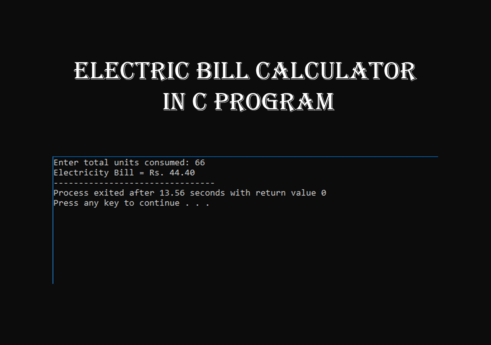## Electric bill calculator in C with source code

Introduction Electric bill calculator is a c programming based project. It generates the total price of electric bill by calculating the unit consumed.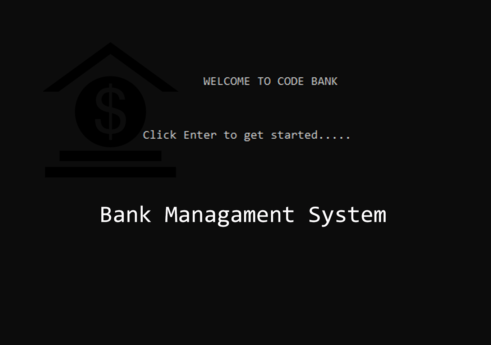## Bank management in C with source code

Introduction Bank management system project is a c programming based project. It has a command-line interface. It is useful for managing a bank account in a bank.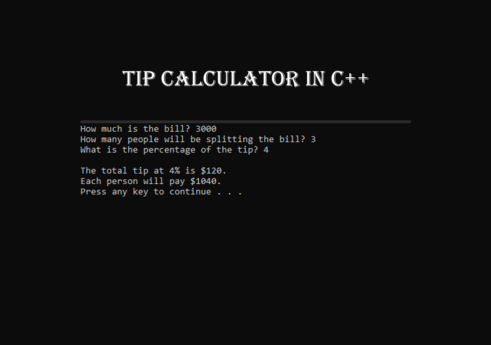## Tip calc in C++ with source code

Introduction Tip calc is a tip calculator based mini project in c plus plus(C++). It is a C++ programming-based project. It is easy to use and implement.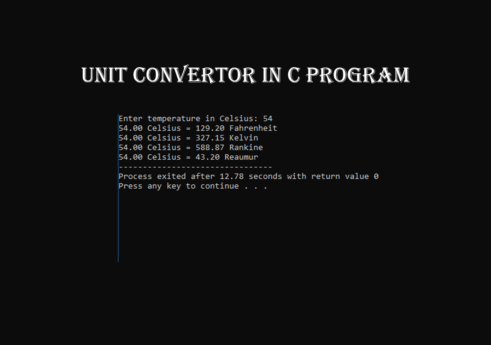## Unit convertor in C program with source code

Introduction Unit convertor is a c programming based project. It converts one single unit into different units. Unit convertor is easy to use.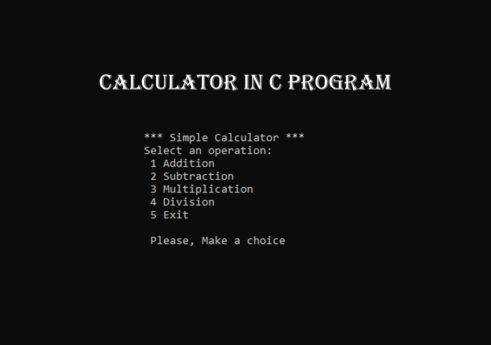## Calculator in C program with source code

Introduction Calculator project is a simple calculator based mini C programming based project. It is easy to use, understand, and implement.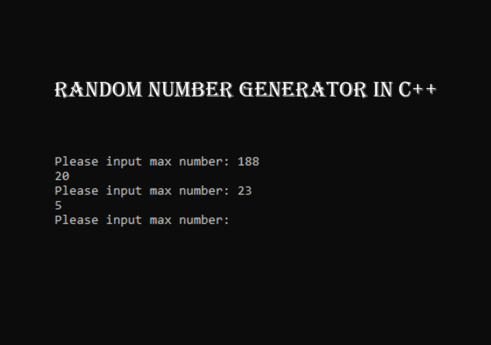## Random number generator in C++

Introduction Random number generator is a mini project in c plus plus(C++). It is a C++ programming-based project.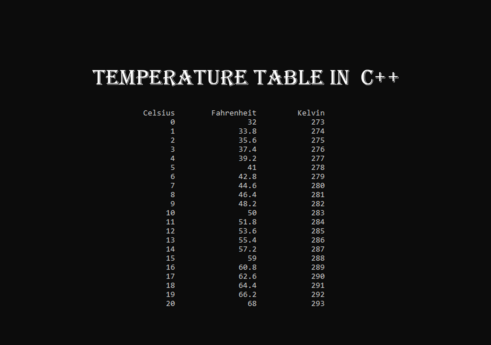## Temperature table in C++ with source code

Introduction Temperature table is a mini project in c plus plus(C++). It is a C++ programming-based project. This is a beginner level project.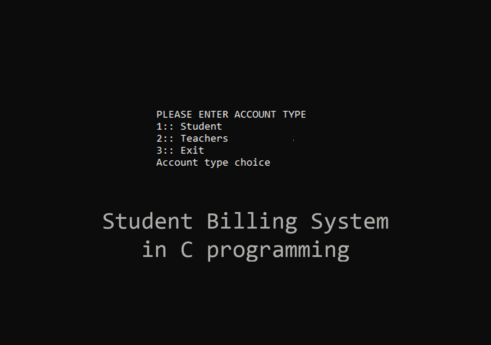## School billing system in C with source code

Introduction School billing system project is a school’s project. It is a c programming-based project. This project deals with activities that happen in school.## Program C

Introduction Program C is the Procedure-oriented programming, machine-independent, and structured programming language. It is very popular and simple which provides low –level access to the memory system.## Department management system

Introduction Department management system project is the concept of the department how it works. It is a c programming based project. It is easy to use.